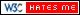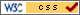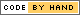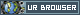# Python Optimization

## Design

It was observed that computing four nearest neighbor for each digit was repetitive and independent task. For example, finding 4 nearest neighbors between input data-set and digit 0 learning data-set did not depend on finding the nearest neighbors for digit 1. Hence, the computation of nearest neighbors for each digit could be handled independently and if possible, could be computed in parallel. The plan was to use all the available cores of the processor to speed-up the computation.

In order to find an effective method to properly utilize all available cores in the system, few experiments were conducted using different modules in python to determine the method which could provide maximum speed-up.

For this experiment, multithreading module available in python was used in order to launch multiple threads from within the program. In order to test the effectiveness of this module, a simple program was written which launched two parallel threads to complete a compute intensive task. The python code for this program is mentioned below. The performance of this python code was analysed using two parameters. First, how effectively the program was able to fire up the available cores on Raspberry-PI. Second, how long did the program take to complete execution of the compute intensive task. On executing the program, it was observed that the python program was not able to utilize the available multi-core system on Raspberry-PI effciently (Figure 1). None of the cores got 100% utilized. In addition, the total execution time observed for this program was 24.9 seconds.

#### Experiment 2: Multi-Processing

To achieve further improvement in execution time and take advantage of multiple-cores on Raspberry-PI, the multiprocessing module of python was used. Multiprocessing is a package that supports spawning processes using an API similar to the threading module. The multiprocessing package offers both local and remote concurrency, effectively side-stepping the Global Interpreter Lock by using sub processes instead of threads. Due to this, the multiprocessing module allows the programmer to fully leverage multiple processors on a given machine . To check the effectiveness of this module, an initial simple code was written which performed the same compute intensive task done by multi-threading program. Two processes of this computation intensive task were created and launched. On observing the core utilization, it was noted that this python code was able to fire-up two different cores and perform parallel computation (Figure 2). Compared to multi-threading program, the execution time of this program reduced by close to 50% to 11.36 seconds. Hence, it was decided to use multi-processing module to achieve higher speed-up for digitrec program.

#### Digitrec Multiprocessing

To develop a generalized digitrec-code using multithreading module was a challenging task. The major hurdle was to make the program adaptable to the system on which it was being executed. The system on which this code was to be executed could have variable number of cores (1, 2, 4 or 8). However, the program should be able to adapt to the environment and launch desired number of processes. For example, launching two processes on a quad-core system would have been under-utilization of resources. At the same time, launching 4 processes on a dual-core system would have resulted in a sub-par performance due to context switches and process-swaps. In order to ensure optimal performance, first the number of cores available on the system was querried with the help of cpu_count() class of multiprocessing module. To determine the number of learning data-sets to be launched in each process, the CPU count was divided by ten. The last process would take care of the remaining number of learning data-sets. The k-nearest neighbor function was also parameterized to ensure its compatibility with the multiprocessing function calls which passed different set of digit learning-sets to parse through. For returning the 4 nearest neighbor distances, a queue was used by each process. Each process pushed the 4 nearest neighbors array of corresponding digits into the queue and the main function de-queued these values in the main function. These arrays were appended in a single array and passed on for digit prediction.

On executing this code in Raspberry-PI, all the four-cores available on the system were fired up. This resulted in 3x speedup in the execution of the code. The execution time reduced from 15 second for the un-optimized version to 5 seconds for the multi-processing version. This ensured that even after signifcant increase in data-set for the digitrec learning database, the total execution time wouldn't execeed few seconds and would provide a real-time performance from the perspective of a user.

## Testing

On executing this code in Raspberry-PI, it was observed that all the four-cores available on the system were fired up for a short period of time (Figure 3). This resulted in 3x speedup in the execution of the code. The execution time reduced from 1.4 second for the un-optimized version to 0.5 seconds for the multi-processing version. This ensured that even after signifcant increase in data-set for the digitrec learning database, the total execution time wouldn't execeed few seconds and would provide a real-time performance from the perspective of a user.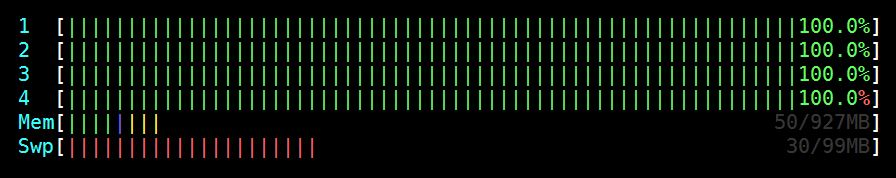## Code Appendix

```# Code developed by Mayank Sen Sharma for ECE5990 Project

from multiprocessing import Process
import time

a = 0
limit = 20000000
while limit > 0:
a = 12323425345*3464758
limit = limit - 1

start_time = time.time()

t.start()
y.start()
t.join()
y.join()

print("\n--- %s seconds ---\n" % (time.time() - start_time))
```

### Multi-Processing Experiment Code

```# Code developed by Mayank Sen Sharma for ECE5990 Project
# Testing Multi-Processing

from multiprocessing import Process
import time

a = 0
limit = 20000000
while limit > 0:
a = 12323425345*3464758
limit = limit - 1

start_time = time.time()

t = Process(target = infinite_loop, args=(5,)) #start( infinite_loop, ("Thread-1",) )
t.start()
y = Process(target = infinite_loop, args=(8,)) #start( infinite_loop, ("Thread-1",) )
y.start()
t.join()
y.join()

print("\n--- %s seconds ---\n" % (time.time() - start_time))
```

### Digitrec Code for launching multiple Processes

```# Developed by Mayank Sen Sharma for ECE5990
# Code for launching multiple processes

# getting number of CPUs
num_cpus = multiprocessing.cpu_count()

# for distribution of distance calculation
step = 10/num_cpus;

# Initializing queuess for function return
ques = [Queue() for i in range(num_cpus)]

# creating multiple processes
proc = [Process(target = k_nearest_neighbor, args=(i*step, step, ques[i])) for i in range(num_cpus - 1)]

proc.append(Process(target = k_nearest_neighbor, args=((num_cpus - 1) * step, 10 - (num_cpus - 1)* step, ques[num_cpus - 1])))

# Starting processes
for i in range(num_cpus):
proc[i].start()

# storing return values from processes
knn = [0 for x in range(num_cpus)]

for i in range(num_cpus):
knn[i] = ques[i].get()

for i in range(num_cpus - 1):
Knn[i*step:(i+1)*step] = knn[i][i*step:(i+1)*step]

Knn[(num_cpus - 1) * step : 10 ] = knn[num_cpus - 1] [(num_cpus - 1) * step : 10 ]
```

## References

 https://docs.python.org/2/library/multiprocessing.html

## Contact

Mayank Sen Sharma (ms3272[at]cornell.edu), Siddharth Mody (sbm99[at]cornell.edu)

Graduate students of Class 2016 at Cornell University

## Ack

Special thanks to Prof. Joseph Skovira and Gautham Ponnu